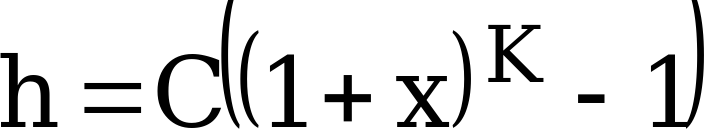## 6 Coding

03.323GPPRelease 98TSUniversal Geographical Area Description (GAD)

## 6.1 Point

The co-ordinates of an ellipsoid point are coded with an uncertainty of less than 3 metres

The latitude is coded with 24 bits: 1 bit of sign and a number between 0 and 223-1 coded in binary on 23 bits. The relation between the coded number N and the range of (absolute) latitudes X it encodes is the following (X in degrees):

except for N=223-1, for which the range is extended to include N+1.

The longitude, expressed in the range -180°, +180°, is coded as a number between -223 and 223-1, coded in 2’s complement binary on 24 bits. The relation between the coded number N and the range of longitude X it encodes is the following (X in degrees):

## 6.2 Uncertainty

A method of describing the uncertainty for latitude and longitude has been sought which is both flexible (can cover wide differences in range) and efficient. The proposed solution makes use of a variation on the Binomial expansion. The uncertainty r, expressed in metres, is mapped to a number K, with the following formula:

with C = 10 and x = 0,1. With 0 £ K £ 127, a suitably useful range between 0 and 1800 kilometres is achieved for the uncertainty, while still being able to code down to values as small as 1 metre. The uncertainty can then be coded on 7 bits, as the binary encoding of K.

Table 1: Example values for the uncertainty Function

 Value of K Value of uncertainty 0 0 m 1 1 m 2 2,.1 m – – 20 57,.3 m – – 40 443 m – – 60 3 km – – 80 20 km – – 100 138 km – – 120 927 km – – 127 1800 km

## Altitude

Altidude is encoded in increments of 1 meter using a 15 bit binary coded number N. The relation between the number N and the range of altitudes a (in metres) it encodes is described by the following equation;

except for N=215-1 for which the range is extended to include all greater values of a.

The direction of altitude is encoded by a single bit with bit value 0 representing height above the WGS84 ellipsoid surface and bit value 1 representing depth below the WGS84 ellipsoid surface.

## Uncertainty Altitude

The uncertainty in altitude, h, expressed in metres is mapped from the binary number K, with the following formula:with C = 45 and x = 0,.025. With 0 £ K £ 127, a suitably useful range between 0 and 990 meters is achieved for the uncertainty altitude,. The uncertainty can then be coded on 7 bits, as the binary encoding of K.

Table 2: Example values for the uncertainty altitude Function

 Value of K Value of uncertainty altitude 0 0 m 1 1,.13 m 2 2,.28 m – – 20 28,.7 m – – 40 75,.8 m – – 60 153,.0 m – – 80 279,.4 m – – 100 486,.6 m – – 120 826,.1 m – – 127 990,.5 m

## Confidence

The confidence by which the position of a target entity is known to be within the shape description, (expressed as a percentage) is directly mapped from the 7 bit binary number K, except for K=0 which is used to indicate ‘no information’, and 100 < K ≤128 which should not be used but may be interpreted as “no information” if received.

## Radius

Inner Rradius is encoded in increments of 5 meters using a 16 bit binary coded number N. The relation between the number N and the range of radius r (in metres) it encodes is described by the following equation;

Except for N=216-1 for which the range is extended to include all greater values of r. This provides a true maximum radius of 327,.675 meters.

The uncertainty radius is encoded as for the uncertainty latitude and longitude.

## Angle

Offset and Included Aangle areis encoded in increments of 21 using an 98 bit binary coded number N in the range 0 to 179. The relation between the number N and the range of offset (ao) and included (ai) angles a (in degrees) it encodes is described by the following equations;

Offset angle (ao)

2 N <= ao < 2 (N+1) Accepted values for ao are within the range from 0 to 359,9…9 degrees.

Included angle (ai)

2 N < ai <= 2 (N+1) Accepted values for ai are within the range from 0,0…1 to 360 degrees.